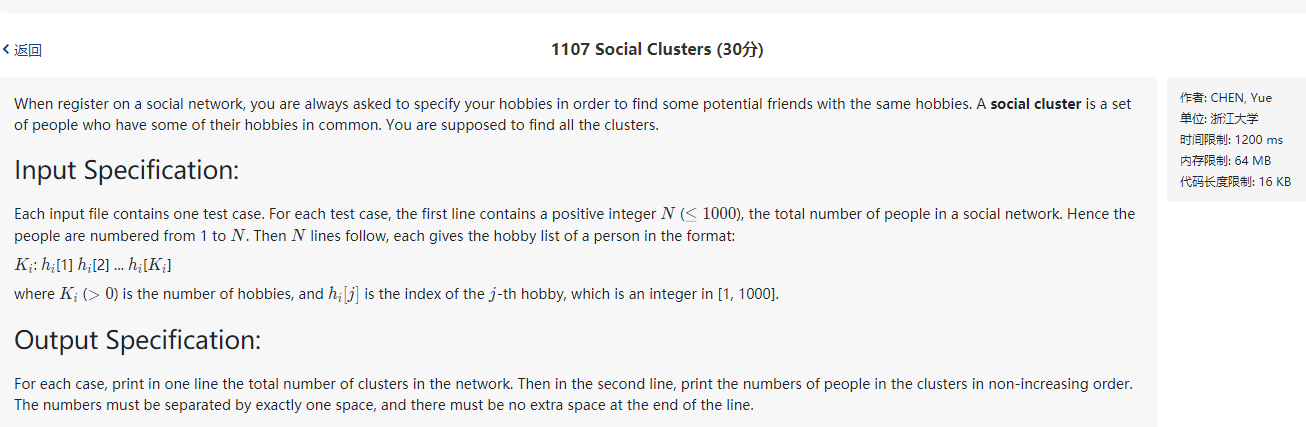``````PAT甲级1107.
``````

# A1107

## 题目## 样例

``````8
3: 2 7 10
1: 4
2: 5 3
1: 4
1: 3
1: 4
4: 6 8 1 5
1: 4
``````

``````3
4 3 1
``````

## 思路和坑点

使用并查集统计不同的社团。对于每一个爱好，假设把数据中的第一个认为是该社团的代表，每次读取数据时，将相同爱好的人合并到同一个并查集（这里并查集的根指向一个负数——其绝对值代表该并查集的元素个数），最后遍历并查集，统计并查集的个数和人数，最后输出结果。

## AC代码

``````#include<iostream>
#include<stdio.h>
#include<stdlib.h>
#include<math.h>
#include<string.h>
#include<algorithm>
#include<map>
#include<unordered_map>
#include<vector>
#include<queue>
#include<stack>
#include<string>
#include<set>
using namespace std;
#define MAXN 1003
int n;
vector<int> hobby(MAXN);        //爱好数组，记录一个喜欢i的人的编号，作为该集团的根
vector<int> v(MAXN);            //并查集 ，初始化为-1
int findfather(int a);          //查询所属并查集的根结点
void Union(int a,int b);        //合并两个并查集（由于此题规模比较小，可以不考虑合并时的大小）
int main(){
#ifdef ONLINE_JUDGE
#else
freopen("1.txt", "r", stdin);
#endif
fill(hobby.begin(),hobby.end(),-1); //初始化hobby数组为-1，表示所有的爱好暂时都没有人
fill(v.begin(),v.end(),-1);         //并查集初始化为-1，表示每一个元素单独构成一个并查集，其中只有自己一个元素
scanf("%d",&n);
for(int i=1;i<=n;i++){              //读入第i个人的爱好
int num;
scanf("%d:",&num);              //第i个人的爱好数量
for(int j=0;j<num;j++){
int h;
scanf("%d",&h);
if(hobby[h]==-1){           //如果是第一个喜欢h活动的人，更新hobby数组，即等同于将这个人作为hobby[h]社团的社长
hobby[h]=i;
}
else
Union(i,findfather(hobby[h]));  //否则把i号人合并进入h爱好的社群
}
}
vector<int> out;                    //out用于输出结果
for(int i=1;i<=n;i++){
if(v[i]<0){                     //如果i是并查集的根则进行统计
out.push_back(v[i]);
}
}
sort(out.begin(),out.end());        //对结果进行排序，由于并查集的根指向负数（其绝对值表示并查集元素个数），从小到大，也就是人数从大到小排序
cout<<out.size()<<'\n';             //输出社团个数
for(int i=0;i<out.size();i++){
if(i) putchar(' ');
printf("%d",-out[i]);           //输出每个社团的人数
}
return 0;
}
int findfather(int a)
{
while(v[a]>0){              //并查集查找根，其中根指向一个负值
a=v[a];
}
return a;
}
void Union(int a,int b)         //合并两个并查集
{
int fa=findfather(a);       //先要找到两个并查集的根
int fb=findfather(b);
if(fa!=fb){                 //如果两个元素不再同一个并查集中，则合并两个并查集
v[fa]+=v[fb];           //可以考虑将小的并查集并入大的并查集中，以减小并查集规模，加快查询速度
v[fb]=fa;
}
}
``````